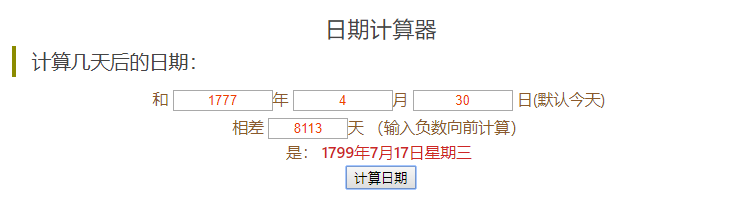# 2013年第四届蓝桥杯C/C++程序设计本科B组省赛 高斯日记(结果填空)

大数学家高斯有个好习惯：无论如何都要记日记。

他的日记有个与众不同的地方，他从不注明年月日，而是用一个整数代替，比如：4210

后来人们知道，那个整数就是日期，它表示那一天是高斯出生后的第几天。这或许也是个好习惯，它时时刻刻提醒着主人：日子又过去一天，还有多少时光可以用于浪费呢？

高斯出生于：1777年4月30日。

在高斯发现的一个重要定理的日记上标注着：5343，因此可算出那天是：1791年12月15日。

高斯获得博士学位的那天日记上标着：8113

请你算出高斯获得博士学位的年月日。#include<stdio.h>

int a={0,31,28,31,30,31,30,31,31,30,31,30,31};
int check(int y)
{
if((y%4==0&&y%100!=0)||(y%400==0)) return 1;
else return 0;
}

int main()
{
int year,month,day;
scanf("%d%d%d",&year,&month,&day);

int y=year,m=month,d=day,sum;
scanf("%d",&sum);

sum+=(d-1);//将日归为1号,天数加到总天数去方便年和月的进位计算
d=1;
//printf("sum===%d\n",sum);
while(sum>=366)
{
if(check(y)) sum-=366;
else sum-=365;
y++;//sum减完之后再加
//printf("sum========%d y========%d\n",sum,y);
}
//printf("y===%d\n",y);
//printf("sum===%d\n",sum);

for(int i=month;i<=12;i++)
{
if(sum<a[i]) break;
sum-=a[i];
if(i==2&&check(y)) sum--;//闰年二月份多减一天
//printf("%d 月减了%d sum=============%d\n",i,a[i],sum);
if(i==12)
{
y++;
i=0;
}
m=i+1;
}

if(sum>0) d+=sum;//如果剩下没有天数则d就是初始值1

printf("year===%d\n",y);
printf("month===%d\n",m);
printf("day===%d\n",d);
return 0;
}